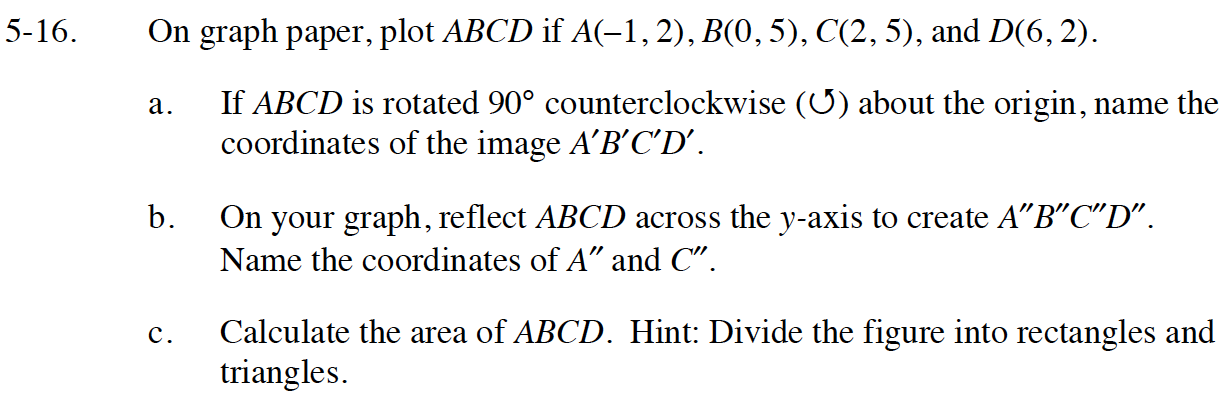Home > CC4 > Chapter 5 > Lesson 5.1.1 > Problem5-16

5-16.
1. On graph paper, plot ABCD if A(–1, 2), B(0, 5), C(2, 5), and D(6, 2). 5-16 HW eTool (Desmos). Homework Help ✎

1. If ABCD is rotated 90° counterclockwise (↺) about the origin, name the coordinates of the image ABCD′.

2. On your graph, reflect ABCD across the y-axis to find ABCD″. Name the coordinates of A″ and C″.

3. Calculate the area of ABCD. Hint: Divide the figure into rectangles and triangles.$\text{Area of a trapezoid }=\frac{1}{2}(b_{1}+b{_{2}})h$

Complete the tables in the eTool below to plot the points, rotate and reflect the shape.
Click on the link at the right to view full version of the eTool: Int1 5-16 HW eTool.